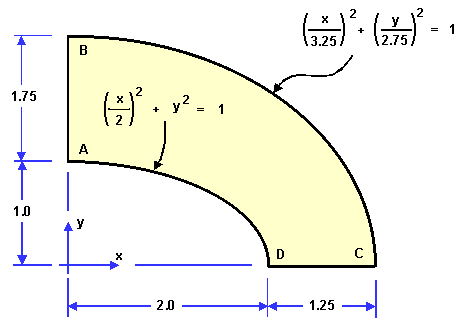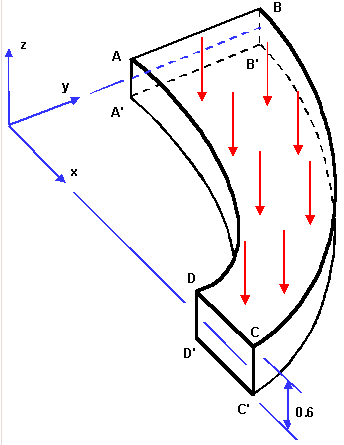# NAFEMS LE10 Benchmarck

This benchmark is extract from the Abaqus Benchmarks Manual.

## 1. Definition

We focus on the LE10 benchmarks in particular.

## 2. Running the case

The command line to run this case is

``mpirun -np 4 feelpp_toolbox_solid --case "github:{path:toolboxes/solid/NAFEMS-LE10}"``

## 3. Data files

The case data files are available in Github here

### 3.1. Geometry

The geometry is given here by :where the thickness is $0.6$.

In addition, we define the point E which is the midpoint of CC' and E' the midpoint of BB'.

### 3.2. Boundary conditions

We set:

• $u_y = 0$ on DCD’C'

• $u_x = 0$ on ABA’B'

• $u_x = u_y = 0$ on BCB’C'

• $\bar{\bar{\varepsilon}}\cdot\mathbf{n}=-1e^6$ on the top surface.

## 4. Inputs

We have the following parameters:

Table 1. Inputs
Name Value

$E$

$210\, GPa$

$\nu$

$0.3$

$\rho$

$7800\, kg/m^2$

## 5. Outputs

We want to compare the value of $\sigma_{yy}$ at the point D. The reference value is $5.38\, MPa$.

## 6. Results

The value of $\sigma_{yy}$ at the point D is $5.53\, MPa$ for $300 000$ dofs, which is $2.78%$ higher than the target.

One possibility to get a more accurate output is to use a mixed formulation, where the stress tensor would also be an unknown.